Technical Information Site of Power Supply Design

2020.12.23 DC/DC

# Parallel Connection of LDOs Using Diodes

Parallel Connections of LDO Linear Regulators

In the previous article, it was indicated that there are two methods of parallel-connecting LDOs. In this article one of these, parallel connections using diodes, is explained.

Parallel Connection of LDOs Using Diodes

We begin by presenting the circuit diagram for parallel connection using diodes. In this connection method, because diodes are present in output paths, the output voltage drops by the amount of the diode forward voltage (hereafter VF). Consequently, some measure such as adding VF back to the LDO setting output voltage must be used in order to obtain the desired output voltage. Moreover, there is some scattering in VF values among diodes, and the value also changes depending on the load current and temperature, so that precision in the output voltage cannot be expected.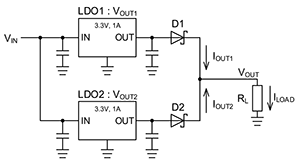Circuit with two LDO outputs connected using diodes

In this circuit also, like the one previously explained, current is supplied from the LDO with the higher output voltage, but because the VF of the diode changes depending on the amount of current flowing, the voltage difference between the two LDOs can be absorbed to some extent. The balancing relationship between the output voltages can be expressed using the following equations.

VOUT1－VF1（IOUT1）＝VOUT2－VF2（IOUT2）

VOUT1：LDO1 output voltage
VOUT2：LDO2 output voltage
VF1（IOUT1）：VF of D1 when current is IOUT1
VF2（IOUT2）：VF of D2 when current is IOUT2

As an example, in a case of LDOs with 3.3 V, 1 A outputs, when the output voltage of LDO1 is 1% higher than that for LDO2, the graph on the right shows the output currents supplied to the load by each of the LDOs.

When the total load current is 1 A, even though the output voltage difference between the LDOs is only 1%, a large difference appears in the fractional currents from the LDOs, which are 0.64 A for LDO1 and 0.36 A for LDO2.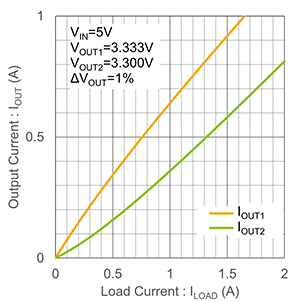Fractional output currents when the difference in output voltages is 1%

Next, the graph on the right indicates the effect on the output currents of the output voltage difference between two LDOs.

This graph shows the ratios of the output currents from the LDOs when the output voltage for LDO1 is higher than that for LDO2. When the output voltages of the two LDOs are exactly the same, the ratios of the output currents are 50% (equal contributions to the output current), but as the output voltage difference increases, the difference in the current ratios grows. For example, if the deviation from the nominal value is +1% for the LDO1 output voltage and -1% for the LDO2 output voltage, then the current output shares are out of balance, at 77% for LDO1 and 23% for LDO2.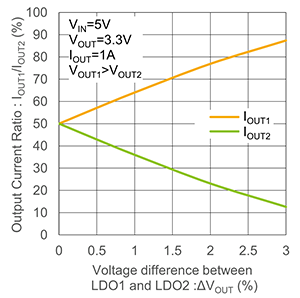Effect on output current ratios of an output voltage difference

The next graph indicates the effect of an output voltage difference between LDOs on the output current of an LDO parallel circuit, when LDOs that each have a maximum recommended output current of 1 A are used.

When there is absolutely no voltage difference between the LDOs, the output currents of the two LDOs are equal, and so an output of 2 A (1 A + 1 A) is possible. As the LDO output voltage difference increases, the current that can be output diminishes. If the output voltage of LDO1 deviates by +1% and that of LDO2 deviates by -1%, when the output current of one of them has reached 1 A, that LDO is in the state of maximum output, and so despite an output current capacity of 2 A, only up to 1.37 A can actually be output.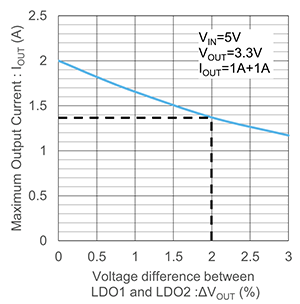Effect on maximum output current of an output voltage difference

Finally, a load regulation graph is presented.

Output voltages drop below the LDO outputs by the VF of the diodes, and as the load current increases the VF values increase, causing a further voltage drop.

In addition to the load regulation provided originally in each LDO, the effect of the diode VF is considerable, and changes in the output voltage are large. Hence, as indicated above, load regulation is not entirely satisfactory.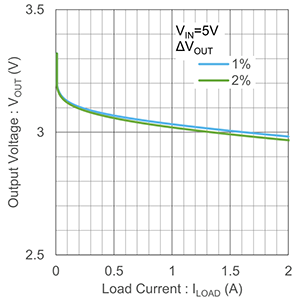Load regulation

In the next article, we will explain the other method of parallel connection, using ballast resistors.

#### Key Points:

・In parallel connections using diodes, because diodes are present in the output paths, the output voltages drop by the amount of the diode VF values, and so measures such as adding a voltage equivalent to VF to the LDO setting output voltage are necessary.

・There is scattering in diode VF values among devices, and the values also change according to the load current and the temperature, and therefore precision in the output voltage (load regulation) cannot be expected.

・Some buffering is achieved by inserting diodes, but in any case, the difference in the output voltages of two LDOs will cause considerable variation in the fractional output currents of the two LDOs.